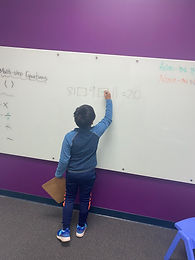## Ms. Briana

### Target 1​

###### Lesson Type:

New

Number Operation

:

Computation

Solve multi-step problems within 100 (addition, subtraction, multiplication, and division).

###### 1:

Solve problems that have more than one operation.

###### 2:

Understand that the presence of parenthesis in an arithmetic equation signals for this part of the problem to be solved first.

###### 3:

Understand when solving a problem that has both addition and subtraction operations that solving starts on the left and continues to the right.

4th

###### Vocabulary:

Operation, Multi-step

Activities:

Students discussed order of operstions and were introduced to order of operations.

Students went around the room solving equations using the order of operations methods they learned.### Home Exploration

###### Guiding Questions:## Absent Students:

elijah

### Target 2

:

###### 1:

Determine the missing arithmetic process from a given equation using understandings of addition, subtraction, multiplication, and division (i.e., if the answer gets larger – than the equation must be using either addition or multiplication).

5th

###### Vocabulary:

Deduce, Properties.

Activities:

Students pulled slips of paper out out of a container for them to deduce the relationship between.

Studentrs took turns pulling and raced to solve and determine relationship.### Home Exploration

###### Guiding Questions:### Target 3

:

###### Vocabulary:

Activities:### Home Exploration